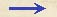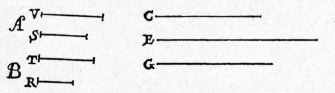Discorsi Propositions 1/05-th-05 Discorsi Proposition1/05-th-05{195} THEOREMA V, PROPOSITIO V. {195} THEOREM V, PROPOSITION VSi duo mobilia aequabili motu ferantur, sint tamen velocitates inaequales, et inaequalia spatia peracta, ratio temporum composita erit ex ratione spatiorum et ex ratione velocitatum contrarie sumptarum. If two particles are moved at a uniform rate, but with unequal speeds, through unequal distances, then the ratio of the time-intervals occupied will be the product of the ratio of the distances by the inverse ratio of the speeds. Sint duo mobilia A, B, sitque velocitas ipsius A ad velocitatem ipsius B ut V ad T; spatia autem peracta sint ut S ad R: dico, rationem temporis quo motum est A, ad tempus quo motum est B, compositam esse ex ratione {10} velocitatis T ad velocitatem V et ex ratione spatii S ad spatium R. Sit ipsius motus A tempus C, et ut velocitas T ad velocitatem V, ita sit tempus C ad tempus E; et cum C sit tempus in quo A cum velocitate V conficit spatium S, sitque ut velocitas T (10) mobilis B ad velocitatem V, ita tempus C ad tempus E, erit tempus E illud in quo mobile B conficeret idem spatium S. Fiat modo ut spatium S ad spatium R, ita tempus E ad tempus G: constat, G esse tempus quo B conficeret spatium R.Et quia ratio C ad G componitur ex rationibus C ad E et E ad G; est autem ratio C ad E eadem cum ratione velocitatum mobilium A, B {20} contrarie sumptarum, hoc est cum ratione T ad V; ratio vero E ad G est eadem cum ratione spatiorum S, R; ergo patet propositum. Let the two moving particles be denoted by A and B, and let the speed of A be to the speed of B in the ratio of V to T; in like manner let the distances traversed be in the ratio of S to R; then I say that the ratio of the time-interval during which the motion of A occurs to the time-interval occupied by the motion of B is the product of the ratio of the speed T to the speed V by the ratio of the distance S to the distance R. Let C be the time-interval occupied by the motion of A, and let the time-interval C bear to a time-interval E the same ratio as the speed T to the speed V. And since C is the time-interval during which A, with speed V, traverses the distance S and since T, the speed of B, is to the speed V, as the time-interval C is to the time-interval E, then E will be the time required by the particle B to traverse the distance S. If now we let the time-interval E be to the time-interval G as the distance S is to the distance R, then it follows that G is the time required by B to traverse the space R.Since the ratio of C to G is the product of the ratios C to E and E to G (while also the ratio of C to E is the inverse ratio of the speeds of A and B respectively, i. e., the ratio of T to V); and since the ratio of E to G is the same as that of the distances S and R respectively, the proposition is proved.Discorsi Propositions 1/05-th-05 Discorsi Proposition1/05-th-05